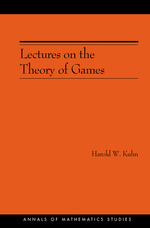# Lectures on the Theory of Games (AM-37)

Harold W. Kuhn
Edition: STU - Student edition
Pages: 120
https://www.jstor.org/stable/j.ctt7t5mk

1. Front Matter
(pp. i-iv)
(pp. v-vi)
3. Author’s Note
(pp. vii-viii)
Harold W. Kuhn
4. Preface
(pp. ix-x)
Harold W. Kuhn
5. Chapter One What Is the Theory of Games?
(pp. 1-4)

The most casual observer of the social behavior of mathematicians will remark that the solitary mathematician is a puzzle-solver while mathematicians together consume an extraordinary amount of time playing games. Coupling this observation with the obvious economic interest of many games of skill and chance, it does not seem strange that the basis for a mathematical theory of games of strategy was created by the mathematician John von Neumann * and elaborated with the economist Oskar Morgenstern . In this series of lectures we shall attempt to present the salient results of this theory.

Although our primary concern will be...

6. Chapter Two Matrix Games
(pp. 5-58)

The well-known game of Matching Pennies provides us with an example of a zero-sum two-person finite game with two moves. (Note that in this game, as in later examples, in order that only one player act at a move, we divide one natural simultaneous move into two consecutive moves.) In the first move,P1chooses the alternative “heads” or “tails,” and in the second move,P2, in ignorance ofP1’s choice, chooses the alternative “heads” or “tails.” After the choices have been made,P2paysP1the amount 1 if they match or -1 if they do not match. We...

7. Chapter Three Extensive Games
(pp. 59-80)

By now the reader will have suspected that matrix games must play an important role in the theory of zero-sum two-person games and that they are much more inclusive than they may have appeared at first sight. Indeed, it may be asserted thatall finite zero-sum two-person games can be reduced to matrix games.The critical reader may justly complain that this statement is meaningless since we have never defined what constitutes a game. Furthermore, although he understands vaguely what is meant by the word “game,” his ideas on the subject are nonmathematical and give no clue as to how...

8. Chapter Four Infinite Games
(pp. 81-104)

The games which have been discussed up to now have been restricted by two conditions mentioned in Chapter 1. These are (1) every move has a finite number of alternatives and (2) every play contains a finite number of moves. It is clear that these restrictions can be relaxed in a wide variety of combinations. Actually, only three general types of infinite games have been studied to any extent: the matrix games with a denumerable number or a continuum of pure strategies  and infinite game trees in which condition (1) above is satisfied . In this chapter, we will...

9. Index
(pp. 105-107)